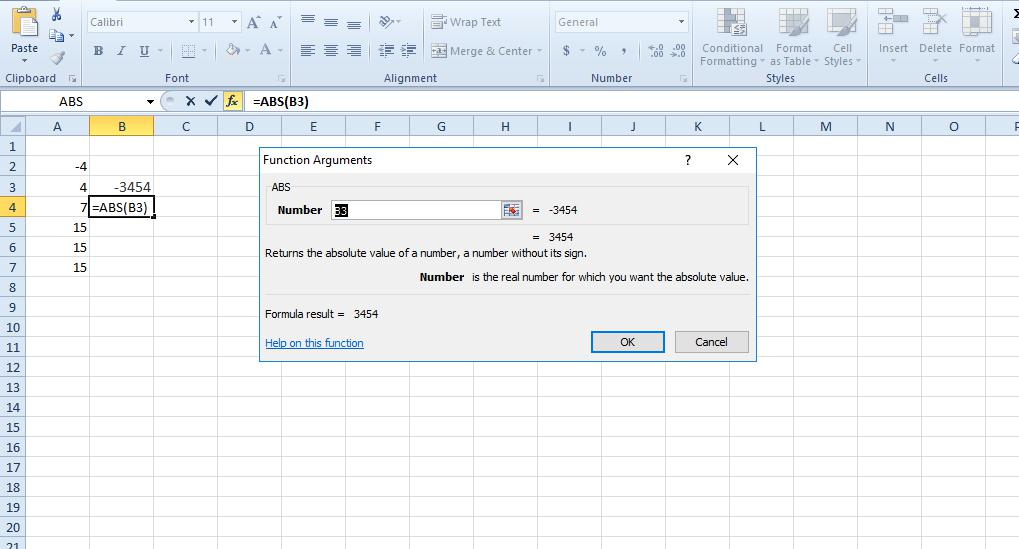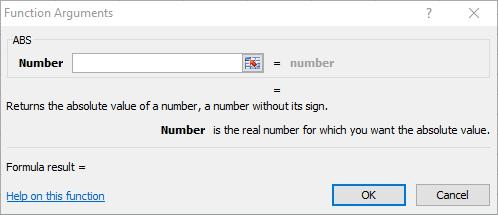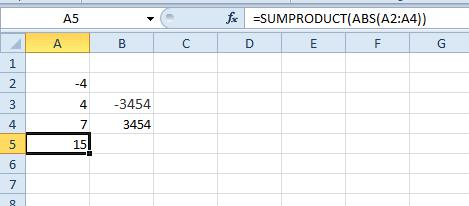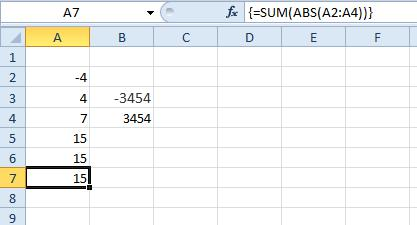# How To Get Absolute Value in Excel

After spending some time with Excel, you might be wondering if there’s an absolute value function in Excel. Thankfully, there is. The absolute value of a number is how far it is from zero. Thus, the absolute value is always a positive number even if the value is negative. For example, the absolute value of -7 is 7. So you don’t really need a spreadsheet to find the absolute values of negative numbers. However, Excel can come in handy for finding the absolute value of a range of positive and negative numbers. This is how you can add absolute values for a data set consisting of negative and positive numbers in Excel.### The ABS Function

ABS is the absolute function you can add to Excel spreadsheets. This is a function that returns the absolute value for a number in a single cell. It’s a basic function that doesn’t add absolute values. The syntax for ABS is: ABS(number).

As an example, open a blank Excel spreadsheet and enter ‘-3454’ in cell B3. Then select cell B4 and press the fx button to open the Insert Function window. Select All from the Or select a category drop-down menu, and click ABS to open the window in the snapshot directly below.Now press the cell reference button for the Number field and select B3. Press the OK button to add the ABS function to spreadsheet. Cell B4 will return a value of 3454 as shown below.You could find the absolute value for a range of cells with this function by adding an ABS column to spreadsheet. Then insert the ABS function in the column’s cells. Enter a =SUM function in a cell at the bottom of the column to add the absolute values.

### Combining ABS with the SUMPRODUCT Function

You can combine ABS with other functions to calculate the absolute value for positive and negative numbers in Excel spreadsheets. SUMPRODUCT is one of the functions that can include ABS to give you the absolute value for a range of positive and negative values.

First, enter some dummy data in your spreadsheet for the SUMPRODUCT function. Enter the values ‘-4,’ ‘4’ and ‘7’ in cells A2, A3 and A4. Select cell A5 and click inside the fx bar. Then input the function ‘=SUMPRODUCT(A2:A4)’ in the fx bar and press the Enter key. That will return 7 in cell A5, which isn’t the absolute value.

To find the absolute value for the range of data, we need to incorporate ABS in the SUMPRODUCT function. So replace the original =SUMPRODUCT (A2:A4) function with =SUMPRODUCT(ABS(A2:A4)). Then A5 will return 15 (4 + 4 + 7) for the cell range as shown directly below.### Find the absolute value with SUMIF

The SUMIF function is one with which you can sum values that meet a specified criteria. As such, you can also find the absolute value for a range of cells added together with SUMIF. The syntax for SUMIF is: SUMIF(range, criteria, [sum_range]).

You can find the absolute value of a range of cells by manually entering the SUMIF function into the fx bar. Select cell A6 and input ‘=SUMIF(A2:A4,”>0″)-SUMIF(A2:A4,”<0″)’ in the function bar. Then when you press Enter, A6 will return the value 15. The function is effectively subtracting all negative numbers from the sum of all the positive values. You can use that function in any spreadsheet by editing the cell references for your sheets.### The SUM Array Formula

Excel array formulas enable users to do multiple calculations for an array (or column of values). Thus, you can also add a SUM array formula to Excel that returns the absolute value of a series of numbers in column or row. You press Ctrl + Shift + Enter to add array formulas to spreadsheets.

The SUM array formula for absolute values is: =SUM(ABS(A2:A4)). Select cell A7 in your spreadsheet, and enter ‘=SUM(ABS(A2:A4))’ in the fx bar. However, don’t press just the Enter key. Instead, you should press the Ctrl + Shift + Enter hotkey after entering the formula in the fx bar. Then the formula will have {} braces around it as shown in the snapshot directly below. This array formula also returns 15 in A7, which is the absolute value for the data entered in cells A2:A4.## How to Multiply Absolute Values in Excel

In case you need to multiply some absolute values in Excel, here’s a quick overview with the PRODUCT and ABS functions. Enter the values ‘-3’ and ‘3’ into cells D2 and D3. Then, in D4, select fx and PRODUCT, your formula should look like this: PRODUCT(D2:D3).The result for this formula is -9, as displayed in cell D4.

Next, in cell D5, select fx and PRODUCT, then ABS and D2:D3. Your formula should be PRODUCT(ABS(D2:D3)). The result will be 9 for this equation, since it uses the ABS function.So, there are a few ways you can find the absolute value for a range of numbers in Excel spreadsheets. SUMIF, SUMPRODUCT, ABS and SUM array are the best functions and formulas for getting absolute value. The Kutools add-on for Excel also includes a Change sign of Values tool that converts negative numbers in the spreadsheet to positive.

Disclaimer: Some pages on this site may include an affiliate link. This does not effect our editorial in any way.

Todays Highlights